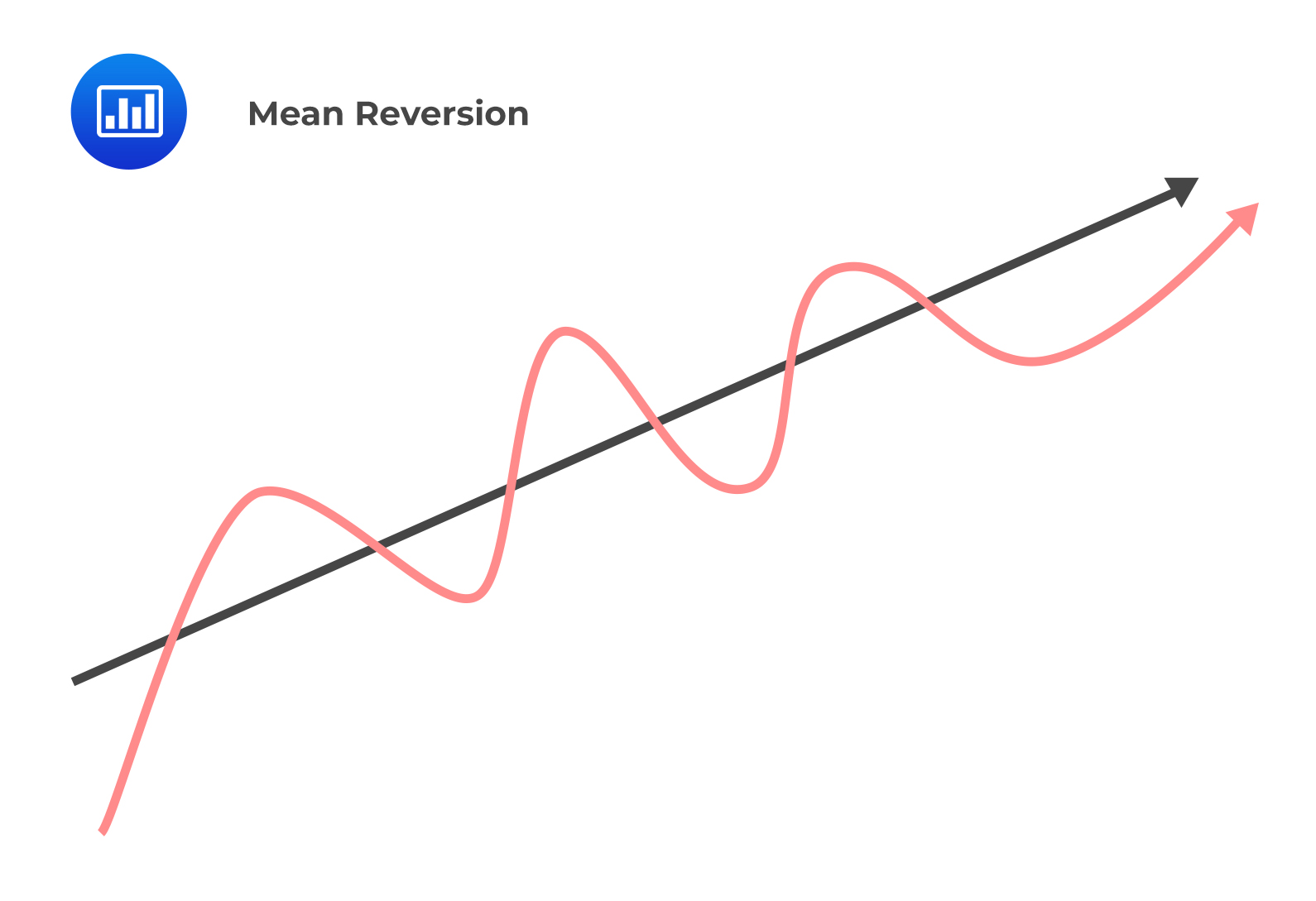# The Mean Reversion

Mean reversion refers to the behavior of a time series to fall when its values are above the mean and rise when they are below the mean. This is illustrated as follows:A mean-reverting time series tends to move towards its long-term mean. The model predicts that the value will stay the same if the time series is currently at its long-term mean.

i.e., $$\hat{\text{x}}_{\text{t}}=\text{x}_{\text{t}-1}$$

Substituting the above in an AR(1) model, we have:

$$\text{x}_{\text{t}}=\text{b}_{0}+\text{b}_{1}\text{x}_{\text{t}-1}=\text{b}_{0}+\text{b}_{1}\text{x}_{\text{t}}$$

The mean-reverting level can be expressed as:

$$\text{x}_{\text{t}}=\text{b}_{0}+\text{b}_{1}\text{x}_{\text{t}}$$

$$\text{x}_{\text{t}}=\frac{\text{b}_{0}}{1-\text{b}_{1}}$$

The value of an AR(1) model will:

Remain the same when $$\text{x}_{\text{t}}=\frac{\text{b}_{0}}{1-\text{b}_{1}}$$

Increase when $$\text{x}_{\text{t}}<\frac{\text{b}_{0}}{1-\text{b}_{1}}$$

Decrease when $$\text{x}_{\text{t}}>\frac{\text{b}_{0}}{1-\text{b}_{1}}$$

#### Example: Mean Reversion

Consider the following output of an AR(1) model.

$$\begin{array}{c|c|c} {}& \textbf{Coefficients} & \textbf{Standard Error} \\ \hline \text{Intercept} & 31.4 & 4.81 \\ \hline \text{Lag 1} & -0.0006827 & 0.0001088\\ \end{array}$$

The mean-reverting level is closest to:

Solution

The mean-reverting level can be expressed as:

$$\text{x}_{\text{t}}=\frac{\text{b}_{0}}{1-\text{b}_{1}}$$

$$\text{x}_{t}=\frac{31.4}{1-(-0.0006827)}=31.39$$

It is worth noting that all covariance stationary time series will have a finite mean-reverting level. This will be discussed in more detail in future learning objectives.

## Question

Consider the following autoregressive model:

$$\text{x}_{t}=0.0407-0.5647\text{x}_{\text{t}-1}$$

The mean-reverting level is closest to:

1. 0.02601.
2. 0.03911.
3. 0.09350.

#### Solution

The mean-reverting level is given by:

$$\text{x}_{\text{t}}=\frac{\text{b}_{0}}{1-\text{b}_{1}}=\frac{0.0407}{1-(-0.5647)}=0.02601$$

LOS 5 (f) Explain mean reversion and calculate a mean-reverting level.

Shop CFA® Exam Prep

Offered by AnalystPrepLevel I
Level II
Level III
All Three Levels
Featured Shop FRM® Exam PrepFRM Part I
FRM Part II
FRM Part I & Part II
Learn with Us

Subscribe to our newsletter and keep up with the latest and greatest tips for success
Shop Actuarial Exams PrepExam P (Probability)
Exam FM (Financial Mathematics)
Exams P & FMGMAT® Complete CourseDaniel Glyn
2021-03-24
I have finished my FRM1 thanks to AnalystPrep. And now using AnalystPrep for my FRM2 preparation. Professor Forjan is brilliant. He gives such good explanations and analogies. And more than anything makes learning fun. A big thank you to Analystprep and Professor Forjan. 5 stars all the way!michael walshe
2021-03-18
Professor James' videos are excellent for understanding the underlying theories behind financial engineering / financial analysis. The AnalystPrep videos were better than any of the others that I searched through on YouTube for providing a clear explanation of some concepts, such as Portfolio theory, CAPM, and Arbitrage Pricing theory. Watching these cleared up many of the unclarities I had in my head. Highly recommended.Nyka Smith
2021-02-18
Every concept is very well explained by Nilay Arun. kudos to you man!2021-02-13